Knowledge

# Series vs. Parallel Circuits: The Complete Breakdown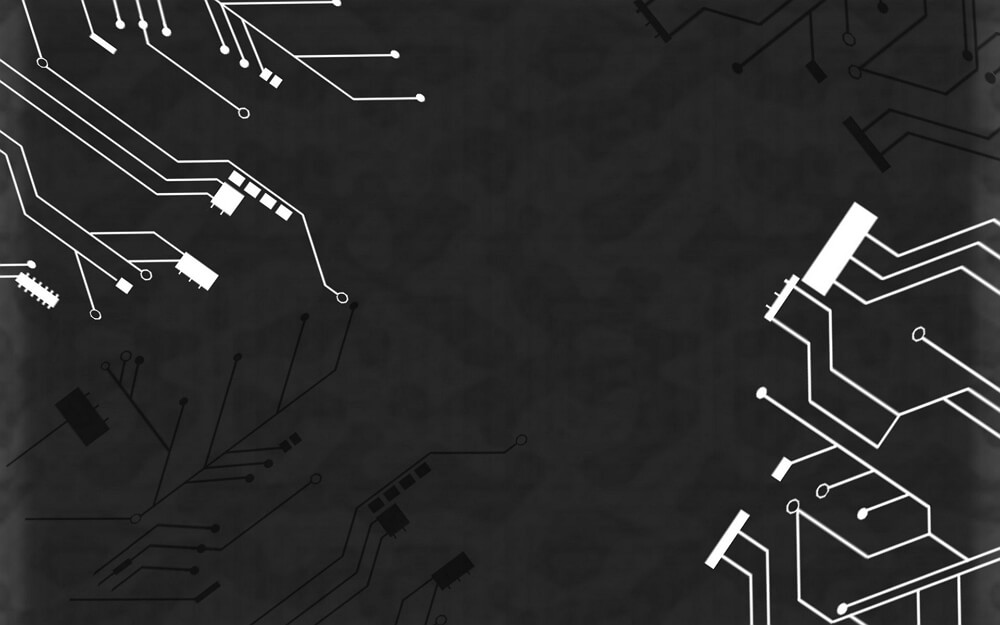## The Basics of Electrical Circuits

Electrical circuits can be arranged in either series or parallel. Series circuits allow for electrons to flow to one or more resistors, which are elements in a circuit that use power from a cell. All of the elements are connected by the same branch. On the other hand, each of the elements in a parallel circuit have their own separate branches.

Here is a breakdown of the common terms and illustrations that you might come across when dealing with series and parallel circuits.

Series: Definition + Illustration Parallel: Definition + Illustration
Current The amount of electricity flowing through a circuit, measured in amps using an ammeter. In this diagram, the current is represented by i.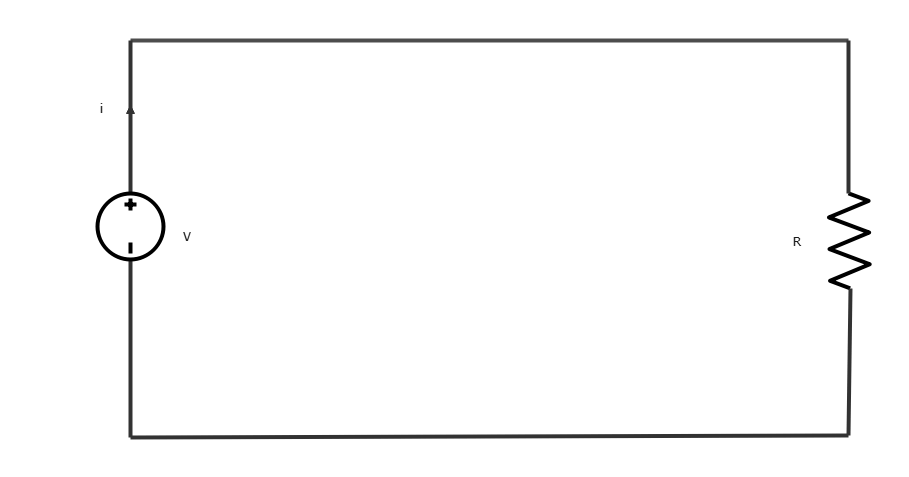The amount of electricity flowing through a circuit, measured in amps using an ammeter. In this diagram, the current is represented by i.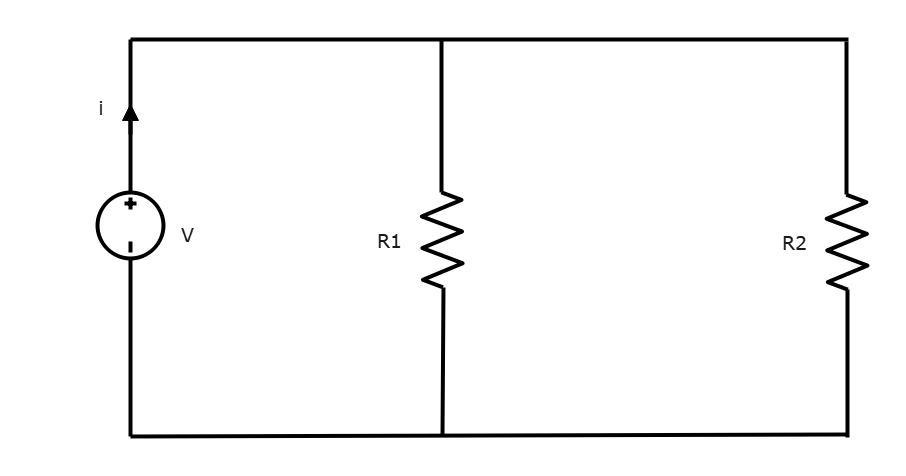Voltage Voltage can be thought of as a force that makes electric charges move in a wire or other kind of conductor. It is measured in volts (v) using a voltmeter.Voltage can be thought of as a force that makes electric charges move in a wire or other kind of conductor. It is measured in volts (v) using a voltmeter.Resistance units Resistance is a measure of the amount of opposition against an electrical circuit’s current flow. Resistance is measured in ohms (Ω).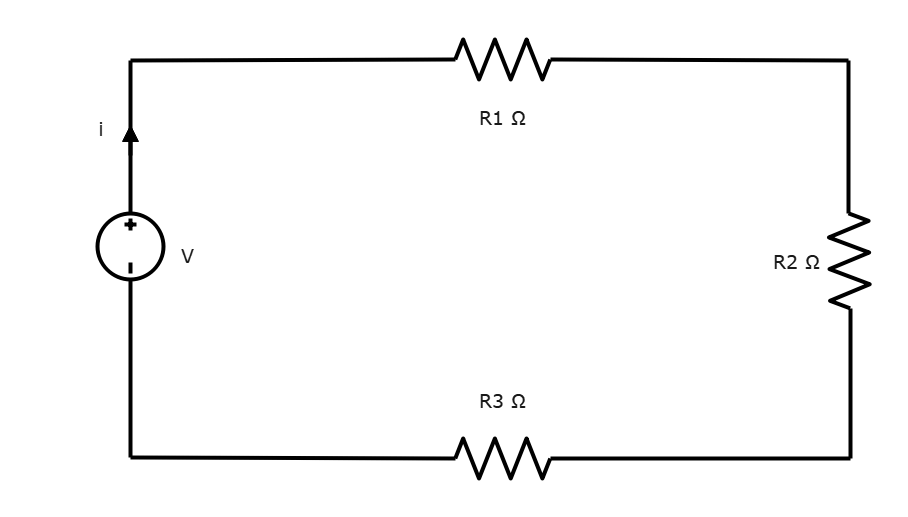Resistance is a measure of the amount of opposition against an electrical circuit’s current flow. Resistance is measured in ohms (Ω).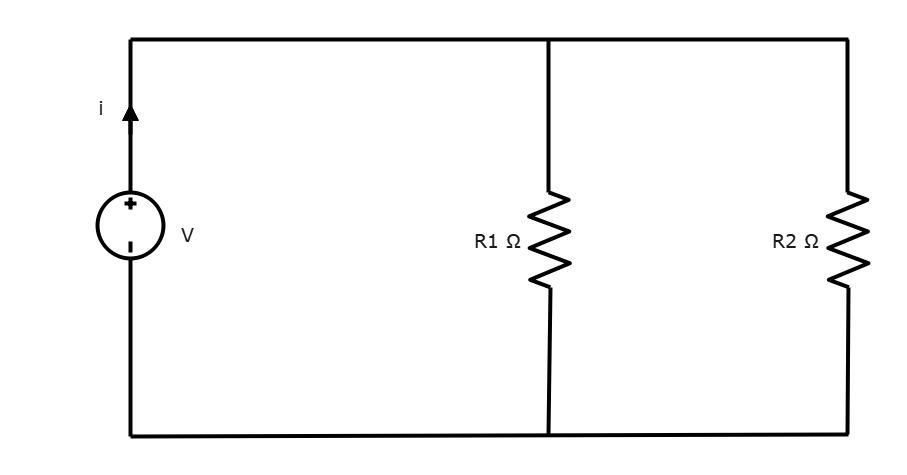Inductors When an electric current flows through an inductor, energy is stored in a magnetic field. It opposes any changes in current that goes through them.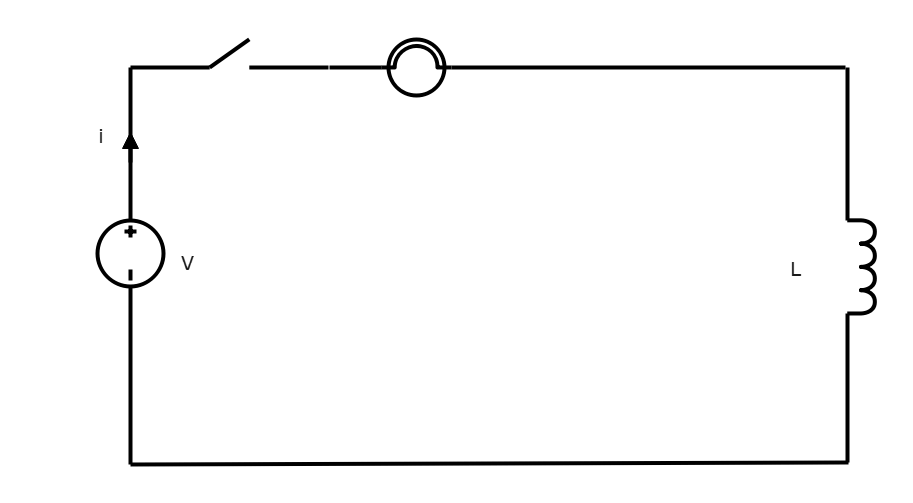When an electric current flows through an inductor, energy is stored in a magnetic field. It opposes any changes in current that goes through them.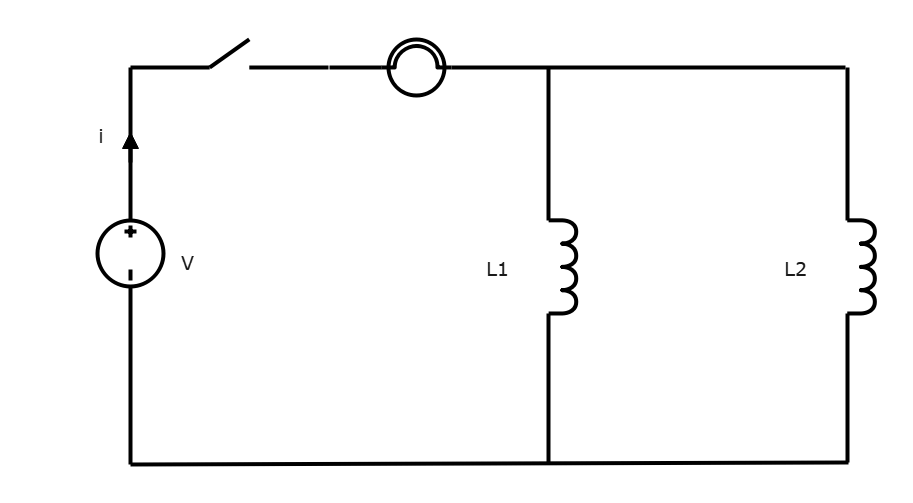Capacitors A capacitor is a passive electronic component that stores electrical energy in an electric field.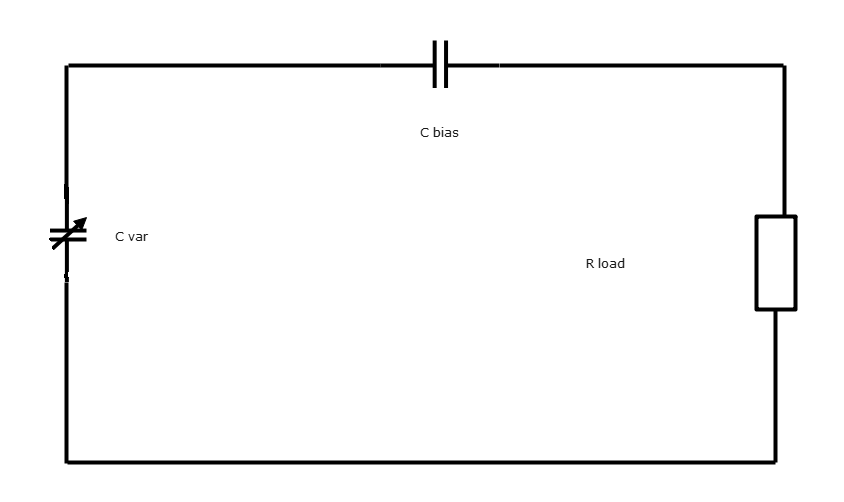A capacitor is a passive electronic component that stores electrical energy in an electric field.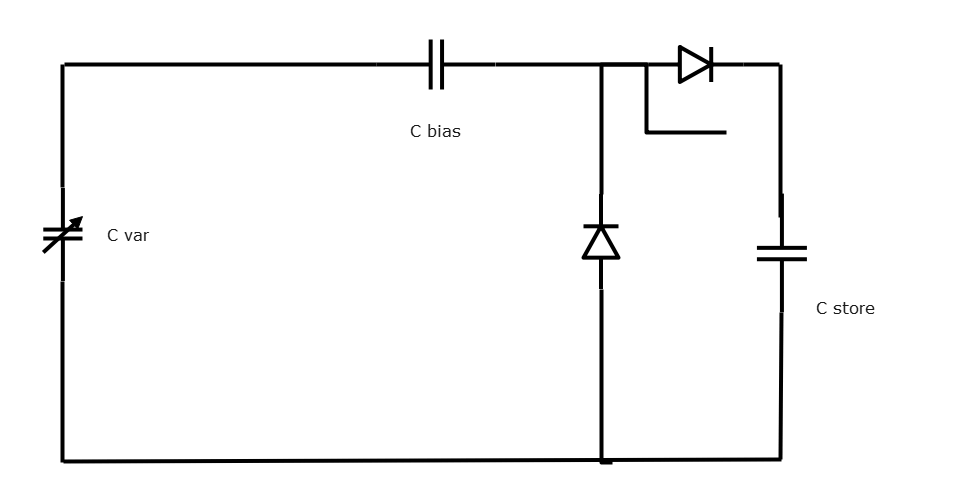Switches A switch is used to control the flow of an electric current in a circuit by inhibiting or initiating its flow.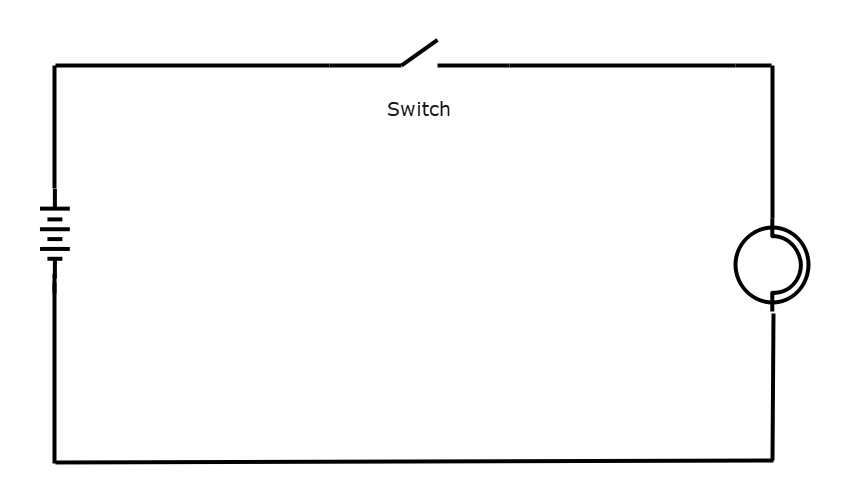A switch is used to control the flow of an electric current in a circuit by inhibiting or initiating its flow.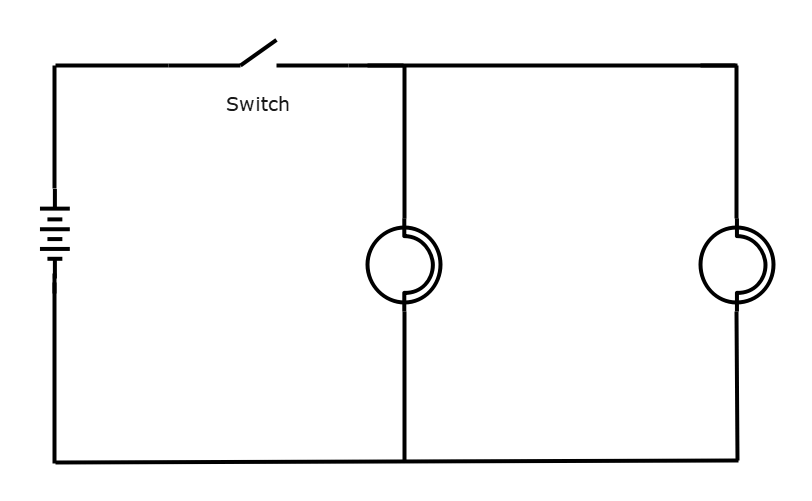Cells and batteries A cell or battery is an energy source that provides the voltage that gets the current flowing in a circuit. It has a positive terminal (+) and a negative terminal (-). The positive terminal is depicted by the longer horizontal line, and the negative terminal is depicted by the shorter horizontal line.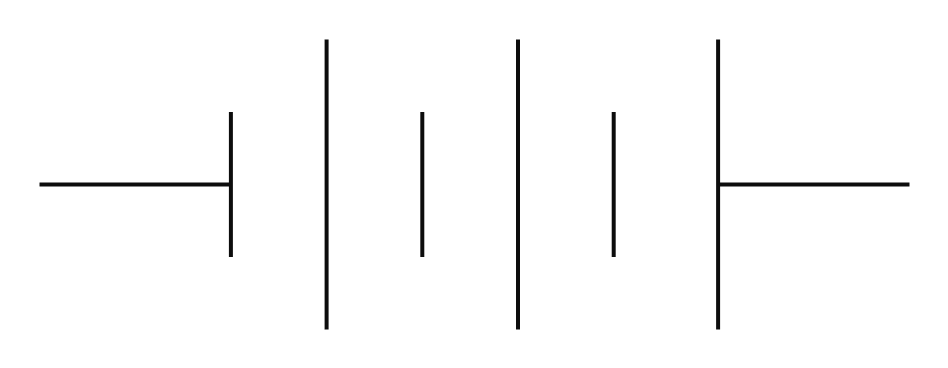A cell or battery is an energy source that provides the voltage that gets the current flowing in a circuit. It has a positive terminal (+) and a negative terminal (-). The positive terminal is depicted by the longer horizontal line, and the negative terminal is depicted by the shorter horizontal line.So now that we’ve covered some of the basics, let’s dive deeper into each of the circuit types.

## EdrawMax

##### All-in-One Diagram Software
Create more than 280 types of diagrams effortlessly
Start diagramming with various templates and symbols easily
• Superior file compatibility: Import and export drawings to various file formats, such as Visio
• Cross-platform supported (Windows, Mac, Linux, Web)Security Verified | Switch to Mac >>

## Series and Parallel Circuits

### Series Circuit

In a series circuit, the resistors are arranged in a chain along one branch. The current going through each resistor is the same. Each component in a series circuit is dependent on each other; if one component was removed, none of the components would turn on.

Series circuits are made up of series connections. All of the components are connected via a single path. The current can only flow through this one path. In this example, there is one path that the current can go through, passing from the cell to the switch and the two bulbs.

An example of a series circuit being used in a real life object is in a lamp. The circuit consists of a power source, a switch, the lamp, and back to the power source. Other examples of series circuits in real life objects include Christmas lights, refrigerators, freezers

• Pros
• Series circuits don’t overheat easily. Dry and flammable objects are less likely to catch on fire if placed near a series circuit.
• Series circuits are very easy to understand and make.
• Adding cells to the circuit will result in greater voltage.
• Cons
• Components of a series circuit are connected to each other. If one breaks or malfunctions, the others won’t turn on. The classic example is Christmas lights.
• The more components there are in a series circuit, the more resistance there will be.

### Parallel Circuit

In a parallel circuit, the current has to pass through at least two different branches. The current will divide among the number of branches. Each resistor will have a constant amount of voltage, but the current passing through each branch may vary.

Parallel circuits consist of parallel connections. In this type of connection, each of the components is connected across each other’s leads, as seen in the diagram below.

One real-life example of a parallel circuit can be found in the lighting and wiring setup in homes. All of the lights are powered by a single power source. If, for example, one light bulb burns out, the power source is still able to send voltage through to the other light bulbs, ensuring that the other appliances can continue to function as normal.

• Pros
• Each component in a parallel circuit receives the same amount of voltage.
• If one component stops working or malfunctions, it won’t affect the other components from working.
• Components can be connected or disconnected from the circuit without impacting the other components.
• Cons
• Parallel circuits are much more difficult to set up. A greater number of wires are needed to construct one.
• Voltage cannot be increased unless the resistance in the parallel circuit is decreased.

### More Examples

Here are two more examples of series and parallel circuits.

Series Circuit Example

Parallel Circuit Example

## Reviews

When it comes to series vs. parallel circuits, each has different electrical properties that you must bear in mind when choosing which one suits your needs best.

• Components in a series circuit form a single path for the current to flow through. This is the simplest kind of circuit, but remember that components are dependent on each other.
• In a parallel circuit, there are at least two branches for the current to flow through. If one component stops working, it won’t affect the others from working.

So why choose EdrawMax? As you can see from the diagrams above, EdrawMax comes equipped with a plethora of symbols and diagrams, including all of the templates that you see above. Give it a try, and find out just how easy creating series and parallel circuit can be! Also, it contains substantial built-in templates that you can use for free, or share your series and parallel circuit templates with others in our template community.

Related Articles
Create a Circuit Diagram with EdrawMax.